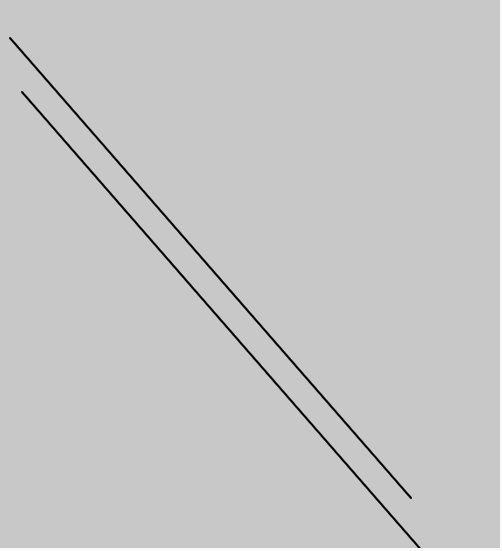# p5.js | createVector() function

The createVector() function in p5.js is used to create the new p5 vector which contains both magnitude and direction. This provides a two or three dimensional vector, specifically a geometric vector.

Syntax:

`createVector([x], [y], [z])`

Parameters: This function accepts three parameters as mentioned above and described below:

• x: This parameter stores the x component of the vector.
• y: This parameter stores the y component of the vector.
• z: This parameter stores the z component of the vector.
• Below programs illustrate the createVector() function in p5.js:

Example 1: This example uses createVector() function to draw a line.

 `function` `setup() { ` ` `  `    ``// Create a Canvas ` `    ``createCanvas(500, 550); ` `} ` ` `  `function` `draw() { ` `    `  `    ``// Vector initislisation ` `    ``// using createVector ` `    ``t1 = createVector(10, 40); ` `    ``t2 = createVector(411, 500); ` `    `  `    ``// Set background color   ` `    ``background(200); ` `    `  `    ``// Set stroke weight ` `    ``strokeWeight(2); ` `    `  `    ``// line using vector ` `    ``line(t1.x, t1.y, t2.x, t2.y); ` `    `  `    ``translate(12, 54); ` `  `  `    ``line(t1.x, t1.y, t2.x, t2.y); ` `} `

Output:Example 2: This example uses createVector() function to draw a circle.

 `function` `setup() { ` ` `  `    ``// Create a Canvas ` `    ``createCanvas(500, 550); ` `} ` `  `  `function` `draw() { ` `    `  `    ``// Vector initislisation  ` `    ``// using createVector ` `    ``t1 = createVector(10, 40); ` `    ``t2 = createVector(41, 50); ` `    `  `    ``// Set background color   ` `    ``background(200); ` `    `  `    ``// Set stroke weight ` `    ``strokeWeight(2); ` `    `  `    ``// Fill yellow ` `    ``fill(``'yellow'``); ` `    `  `    ``// ellispe using vector ` `    ``ellipse(t1.x*millis() / 1000 * 20, ` `            ``t1.y, t2.x+100, t2.y+100);  ` `} `

Output:My Personal Notes arrow_drop_upCheck out this Author's contributed articles.

If you like GeeksforGeeks and would like to contribute, you can also write an article using contribute.geeksforgeeks.org or mail your article to contribute@geeksforgeeks.org. See your article appearing on the GeeksforGeeks main page and help other Geeks.

Please Improve this article if you find anything incorrect by clicking on the "Improve Article" button below.

Article Tags :

Be the First to upvote.

Please write to us at contribute@geeksforgeeks.org to report any issue with the above content.